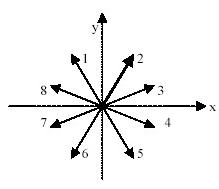# Problem: 1. Find the magnitude of the impulse J delivered to the particle. Express your answer in terms of m and v. Use three significant figures in the numerical coefficient. 2. Which of the vectors below best represents the direction of the impulse vector J?

###### FREE Expert Solution

Impulse:

$\overline{){\mathbf{J}}{\mathbf{=}}{\mathbf{m}}{\mathbf{∆}}{\mathbf{v}}}$

1.

J = m(vf - v0)

The initial velocity, v0 = v i

The final velocity, vf = 2vj###### Problem Details

1. Find the magnitude of the impulse J delivered to the particle. Express your answer in terms of m and v. Use three significant figures in the numerical coefficient.

2. Which of the vectors below best represents the direction of the impulse vector J?